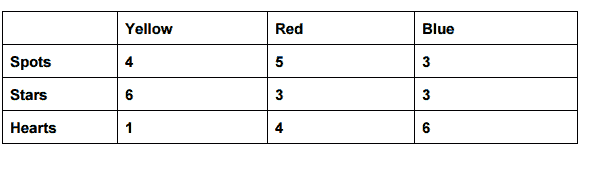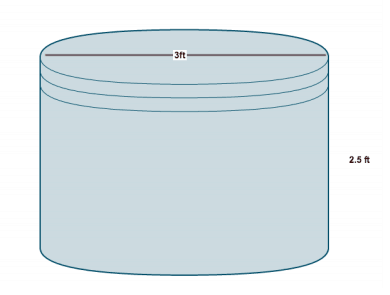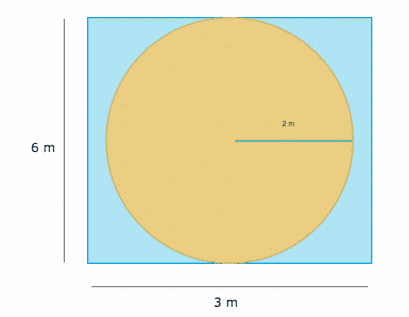# GED Math Practice Test 2 – GED Practice Tests & Online Classes

GED Math Practice Test 2 to help you pass your GED Exam with high scores. Try our free GED Practice Tests and GED Classes. Start Studying Today!

## Question 1 | GED Math Practice Test 2

Question 1 of 15 (click ‘Next Page’ at the bottom right of the page to go on to the next question.)

5 % Complete

A. 99
B. 49
C. 59
D. 109

Question 2 of 15

10 % Complete

A. 12 and 14
B. 12 and 16
C. 18 and 22
D. 22 and 24

Question 3 of 15

16 % Complete

A. \$32
B. \$60
C. \$110
D. \$128

Question 4 of 15

23 % Complete

A. \$10.50
B. \$7.50
C. \$7.00
D. \$12.00

## Question 5 | GED Math Practice Test 2

Question 5 of 15

30 % Complete

### Solve the inequality: y -­ 9 > 18

A. y < 27 B. y > 27
C. y = 27
D. y = 9

## Question 6 | GED Math Practice Test 2

Question 6 of 15

37 % Complete

### Peter went to the hardware store with \$10. He needs to purchase some light plate and outlet covers. Here are the prices at the store:

Light plate covers: \$0.75
Outlet plate covers: \$1.25

He has enough money to purchase which set of item below?

A. 7 light plate covers and 4 outlet plate covers
B. 6 light plate covers and 6 outlet plate covers
C. 6 light plate covers and 4 outlet plate covers
D. 5 light plate covers and 6 outlet plate covers

## Question 7 | GED Math Practice Test 2

Question 7 of 15

44 % Complete

### What is the slope of the line?A. ­2
B. 2
C. ½
D. 7

## Question 8 | GED Math Practice Test 2

Question 8 of 15

51 % Complete

### The table below shows the number of bouncy ball possibilities at an arcade. Each of the bouncy balls have one color and one design. If a red bouncy ball is chosen at random, what is the possibility that it will have stars on it?A. ¼
B. ½
C. 33%
D. 5/12

Question 9 of 15

58 % Complete

A. Thursday
B. Wednesday
C. Tuesday
D. Monday

## Question 10 | GED Math Practice Test 2

Question 10 of 15

65 % Complete

### Taylor is trying to fill an old fire pit in his front yard with dirt. How much dirt will he need to fill the entire pit? Round up to the nearest whole number.A. 16 ft³
B. 18 ft³
C. 20 ft³
D. 21 ft³

## Question 11 | GED Math Practice Test 2

Question 11 of 15

72 % Complete

A. 6 km
B. 12 km
C. 7 km
D. 14 km

## Question 12 | GED Math Practice Test 2

Question 12 of 15

79 % Complete

A. 12 ft²
B. 9 ft²
C. 6 ft²
D. 3ft²

## Question 13 | GED Math Practice Test 2

Question 13 of 15

86 % Complete

### What is the area of the blue space below?A. 2.5 m2
B. 3.9m2
C. 5.5 m2
D. 7.5 m2

## Question 14 | GED Math Practice Test 2

Question 14 of 15

93 % Complete

A. \$172.22
B. \$206.20
C. \$138.89
D. \$105.56

## Question 15 | GED Math Practice Test 2

Question 15 of 15

100 % Complete

A. \$1.69
B. \$1.96
C. \$2.04
D. \$2.06